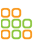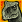# CAN with M4-Core

cancel
Showing results for
Did you mean:
SOLVED

## CAN with M4-Core

1,395 ViewsContributor II

Hi!

I'm trying to make the FlexCAN-module work with the M4-core of my Cosmic+ Board running Phytec`s phyCORE-Vybrid.

The SoM's Freescale version number is:

PVF61GS151CMK50

Test REV C3

1N02G

XAA1308D

I know, that right now the FlexCAN-module is not supported by MQX/Linux (yet?). Are there any plans to support the CAN-module?

Using the Board within CAN-applications is one of the main reasons why I chose the Vybrid. That is why it is very important for me to make the CAN work...

So, what I did so far is trying to initialize the FlexCAN by accessing the corresponding registers "on my own".

Somehow the Vybrid is not able to leave the "Freeze Mode".

This is my function for init-process:

```void can_init(void)
{
struct CAN_MemMap *can0dev;
can0dev = CAN0_BASE_PTR;
/* initialization of CAN RX pinš*/
/* initialization of CAN TX pin */
/* CCM_CCGR2: CG8=2 */
CCM_CCGR2 = (uint32_t)((CCM_CCGR2 & (uint32_t)~(uint32_t)(
CCM_CCGR2_CG8(0x01)
)) | (uint32_t)(
CCM_CCGR2_CG8(0x02)
));
/* IOMUXC_PTB14: ??=0,??=0,??=0,??=0,??=0,??=0,??=0,??=0,??=0,MUX_MODE=1,??=0,??=0,??=0,??=0,??=0,??=0,OBE=0,IBE=0 */
IOMUXC_PTB14 = (uint32_t)((IOMUXC_PTB14 & (uint32_t)~(uint32_t)(
IOMUXC_RGPIO_MUX_MODE(0x06) |
0xFF8FC000U
)) | (uint32_t)(
IOMUXC_RGPIO_MUX_MODE(0x01)
));
/* IOMUXC_PTB15: ??=0,??=0,??=0,??=0,??=0,??=0,??=0,??=0,??=0,MUX_MODE=1,??=0,??=0,??=0,??=0,??=0,??=0,OBE=0,IBE=0 */
IOMUXC_PTB15 = (uint32_t)((IOMUXC_PTB15 & (uint32_t)~(uint32_t)(
IOMUXC_RGPIO_MUX_MODE(0x06) |
0xFF8FC000U
)) | (uint32_t)(
IOMUXC_RGPIO_MUX_MODE(0x01)
));

CAN0_MCR |= CAN_MCR_MDIS_MASK;       /* Disable CAN module */
/*XTAL Clock*/
/* CAN0_CTRL1: PRESDIV=0,RJW=0,PSEG1=0,PSEG2=0,BOFFMSK=0,ERRMSK=0,CLKsrc=0,LPB=0,TWRNMSK=0,RWRNMSK=0,??=0,??=0,SMP=0,BOFFREC=0,TSYN=0,LBUF=0,LOM=0,PROPSEG=0 */
CAN0_CTRL1 = CAN_CTRL1_PRESDIV(0x00) |
CAN_CTRL1_RJW(0x00) |
CAN_CTRL1_PSEG1(0x00) |
CAN_CTRL1_PSEG2(0x00) |
CAN_CTRL1_PROPSEG(0x00);
/* CAN0_MCR: MDIS=0,SOFTRST=1 */
CAN0_MCR = (uint32_t)((CAN0_MCR & (uint32_t)~(uint32_t)(
)) | (uint32_t)(
));                       /* Soft Reset */
while (CAN_PDD_GetSoftResetState(CAN0_BASE_PTR) == CAN_PDD_NOT_RESET){} /* Wait for Soft reset Acknowledge */
while (CAN_PDD_GetFreezeAck(CAN0_BASE_PTR) == CAN_PDD_NOT_FREEZE){} /* Wait for entering the freeze mode */
/* CAN0_MCR: WRNEN=1,SRXDIS=1,MAXMB&=~0x0E,MAXMB|=1 */
CAN0_MCR = (uint32_t)((CAN0_MCR & (uint32_t)~(uint32_t)(
CAN_MCR_MAXMB(0x0E)
)) | (uint32_t)(
CAN_MCR_MAXMB(0x01)
));                       /* MCR reg. Settings */
/* CAN0_CTRL1: PRESDIV|=5,PSEG1|=3,PSEG2|=1,BOFFMSK=1,ERRMSK=1,TWRNMSK=1,RWRNMSK=1,LBUF=1 */
CAN0_CTRL1 |= CAN_CTRL1_PRESDIV(0x05) |
CAN_CTRL1_PSEG1(0x03) |
CAN_CTRL1_PSEG2(0x01) |
CAN_CTRL1_LBUF_MASK;   /* Setting CTRL1 register */
/* CAN0_CTRL2: RRS=1 */
CAN0_CTRL2 |= CAN_CTRL2_RRS_MASK;    /* Setting CTRL2 register */
/* CAN0_MECR: ECRWRDIS=0 */
CAN0_MECR &= (uint32_t)~(uint32_t)(CAN_MECR_ECRWRDIS_MASK); /* Enable writing into Memory error control register */
/* CAN0_MECR: ECRWRDIS=1,ECCDIS=1 */
CAN0_MECR |= (CAN_MECR_ECRWRDIS_MASK | CAN_MECR_ECCDIS_MASK); /* Disable memory error detection and correction */

/* Initialize the message buffer 0 - Rx */
/* CAN0_CS0: ??=0,??=0,??=0,??=0,CODE=4,??=0,SRR=0,IDE=0,RTR=0,DLC=0,TIME_STAMP=0 */
CAN0_CS0 = (CAN_CS_CODE(0x04) | CAN_CS_DLC(0x00) | CAN_CS_TIME_STAMP(0x00));

/* CAN0_ID0: PRIO=0,STD=0x07FF,EXT=0 */
CAN0_ID0 = (CAN_ID_PRIO(0x00) | CAN_ID_STD(0x07FF) | CAN_ID_EXT(0x00));
/* CAN0_WORD00: DATA_BYTE_0=0,DATA_BYTE_1=0,DATA_BYTE_2=0,DATA_BYTE_3=0 */
CAN0_WORD00 = CAN_WORD0_DATA_BYTE_0(0x00) |
CAN_WORD0_DATA_BYTE_1(0x00) |
CAN_WORD0_DATA_BYTE_2(0x00) |
CAN_WORD0_DATA_BYTE_3(0x00);
/* CAN0_WORD10: DATA_BYTE_4=0,DATA_BYTE_5=0,DATA_BYTE_6=0,DATA_BYTE_7=0 */
CAN0_WORD10 = CAN_WORD1_DATA_BYTE_4(0x00) |
CAN_WORD1_DATA_BYTE_5(0x00) |
CAN_WORD1_DATA_BYTE_6(0x00) |
CAN_WORD1_DATA_BYTE_7(0x00);
/* Initialize the message buffer 1 - Tx */
/* CAN0_CS1: ??=0,??=0,??=0,??=0,CODE=8,??=0,SRR=0,IDE=0,RTR=0,DLC=0,TIME_STAMP=0 */
CAN0_CS1 = (CAN_CS_CODE(0x08) | CAN_CS_DLC(0x00) | CAN_CS_TIME_STAMP(0x00));
/* CAN0_ID1: PRIO=0,STD=0,EXT=0 */
CAN0_ID1 = (CAN_ID_PRIO(0x00) | CAN_ID_STD(0x00) | CAN_ID_EXT(0x00));
/* CAN0_WORD01: DATA_BYTE_0=0,DATA_BYTE_1=0,DATA_BYTE_2=0,DATA_BYTE_3=0 */
CAN0_WORD01 = CAN_WORD0_DATA_BYTE_0(0x00) |
CAN_WORD0_DATA_BYTE_1(0x00) |
CAN_WORD0_DATA_BYTE_2(0x00) |
CAN_WORD0_DATA_BYTE_3(0x00);
/* CAN0_WORD11: DATA_BYTE_4=0,DATA_BYTE_5=0,DATA_BYTE_6=0,DATA_BYTE_7=0 */
CAN0_WORD11 = CAN_WORD1_DATA_BYTE_4(0x00) |
CAN_WORD1_DATA_BYTE_5(0x00) |
CAN_WORD1_DATA_BYTE_6(0x00) |
CAN_WORD1_DATA_BYTE_7(0x00);
}

```

Some parts are adapted by the CAN-Driver provided by Processor Experts software.

Has anybody ideas? Can someone help me, please?

Maybe Freescale support?

If you need further information, let my know and I will provide!

Thank you very much!

Manuel

Labels (3)

• ### VF6xx

Tags (5)
1 Solution
716 ViewsSenior Contributor II

Hello Manuel,

I think the logic in line 50 might be incorrect here. If the controller waits in the for loop at line 50 forever, then CAN_MCR_FRZACK_MASK is indeed enabled in CAN0_MCR - which should be the intention, so the configuration bits for CAN0 can be set. So, I think you would want to use the following line for line 50 instead:

This is similar to line 47 in the original code snippet you sent.

Thanks,

Timesys Support

9 Replies
716 ViewsSenior Contributor IV

No software can be an expert in anything... Did expert generate this code for Vybrid? CSCDR2 register setup is missing, CCGR setup is wrong (CCGR0 CG2 clock gate for FlexCAN0, not CCGR2 CG8). Vybrid RM is better expert, you should start there.

Edward

716 ViewsContributor II

The above code is almost exactly what PE generated for the related MV61... modell (same registers etc.), not for Vybrid.

I started with the VybridRM, but there were even more registers I did not think of!

So I tried to generate an example, that I wanted to adapt...

Ok, so I will try to setup the CSCDR2 register and take the right clock source (CCGR0 CG2).

Manuel

EDIT:

According to VybridRM (Rev. 5, 07/2013), page 669 CCGR0 CG0 is the right clock gate for FlexCAN0, right?

CGR2 CG8 is for IOMUX Controller, which I need. Am I right?

716 ViewsSenior Contributor II

Hello Manuel,

Yes, CCGR0[CG0] is the clock gate for FlexCAN0, and CCGR2[CG8] for the IOMUX Controller. You can use CGR mode 11b in order to have the clock on during all modes except stop mode. If you are still experiencing issues, you can try writing 0xFFFFFFFF to the CCGRx registers for debug purposes.

Thanks,

Timesys Support

716 ViewsContributor II

Ok, I tried what you suggested, but nothing has changed.

This is what I have got so far:

```void can_init(void)
{
CCM_CCGR0  = 0xFFFFFFFF;
CCM_CCGR1  = 0xFFFFFFFF;
CCM_CCGR2  = 0xFFFFFFFF;
CCM_CCGR3  = 0xFFFFFFFF;
CCM_CCGR4  = 0xFFFFFFFF;
CCM_CCGR5  = 0xFFFFFFFF;
CCM_CCGR6  = 0xFFFFFFFF;
CCM_CCGR7  = 0xFFFFFFFF;
CCM_CCGR8  = 0xFFFFFFFF;
CCM_CCGR9  = 0xFFFFFFFF;
CCM_CCGR10 = 0xFFFFFFFF;
CCM_CCGR11 = 0xFFFFFFFF;

/* IOMUXC_PTB14: ??=0,??=0,??=0,??=0,??=0,??=0,??=0,??=0,??=0,MUX_MODE=1,??=0,??=0,??=0,??=0,??=0,??=0,OBE=0,IBE=0 */
IOMUXC_PTB14 = (uint32_t)((IOMUXC_PTB14 & (uint32_t)~(uint32_t)(
IOMUXC_RGPIO_MUX_MODE(0x06) |
0xFF8FC000U
)) | (uint32_t)(
IOMUXC_RGPIO_MUX_MODE(0x01)
));
/* IOMUXC_PTB15: ??=0,??=0,??=0,??=0,??=0,??=0,??=0,??=0,??=0,MUX_MODE=1,??=0,??=0,??=0,??=0,??=0,??=0,OBE=0,IBE=0 */
IOMUXC_PTB15 = (uint32_t)((IOMUXC_PTB15 & (uint32_t)~(uint32_t)(
IOMUXC_RGPIO_MUX_MODE(0x06) |
0xFF8FC000U
)) | (uint32_t)(
IOMUXC_RGPIO_MUX_MODE(0x01)
));

CAN0_MCR |= CAN_MCR_MDIS_MASK;      /* Disable CAN module */
/*XTAL Clock*/
/* CAN0_CTRL1: PRESDIV=0,RJW=0,PSEG1=0,PSEG2=0,BOFFMSK=0,ERRMSK=0,CLKsrc=0,LPB=0,TWRNMSK=0,RWRNMSK=0,??=0,??=0,SMP=0,BOFFREC=0,TSYN=0,LBUF=0,LOM=0,PROPSEG=0 */
CAN0_CTRL1 = CAN_CTRL1_PRESDIV(0x00) |
CAN_CTRL1_RJW(0x00) |
CAN_CTRL1_PSEG1(0x00) |
CAN_CTRL1_PSEG2(0x00) |
CAN_CTRL1_PROPSEG(0x00);
/* CAN0_MCR: MDIS=0,SOFTRST=1 */
CAN0_MCR = (uint32_t)((CAN0_MCR & (uint32_t)~(uint32_t)(
)) | (uint32_t)(
));                      /* Soft Reset */
while (!(CAN0_MCR & CAN_MCR_SOFTRST_MASK)){} // wait for reset

/* CAN0_MCR: WRNEN=1,SRXDIS=1,MAXMB&=~0x0E,MAXMB|=1 */
CAN0_MCR = (uint32_t)((CAN0_MCR & (uint32_t)~(uint32_t)(
CAN_MCR_MAXMB(0x0E)
)) | (uint32_t)(
CAN_MCR_MAXMB(0x01)
));                      /* MCR reg. Settings */
/* CAN0_CTRL1: PRESDIV|=5,PSEG1|=3,PSEG2|=1,BOFFMSK=1,ERRMSK=1,TWRNMSK=1,RWRNMSK=1,LBUF=1 */
CAN0_CTRL1 |= CAN_CTRL1_PRESDIV(0x05) |
CAN_CTRL1_PSEG1(0x03) |
CAN_CTRL1_PSEG2(0x01) |
CAN_CTRL1_LBUF_MASK;  /* Setting CTRL1 register */
/* CAN0_CTRL2: RRS=1 */
CAN0_CTRL2 |= CAN_CTRL2_RRS_MASK;    /* Setting CTRL2 register */
/* CAN0_MECR: ECRWRDIS=0 */
CAN0_MECR &= (uint32_t)~(uint32_t)(CAN_MECR_ECRWRDIS_MASK); /* Enable writing into Memory error control register */
/* CAN0_MECR: ECRWRDIS=1,ECCDIS=1 */
CAN0_MECR |= (CAN_MECR_ECRWRDIS_MASK | CAN_MECR_ECCDIS_MASK); /* Disable memory error detection and correction */

/* Initialize the message buffer 0 - Rx */
/* CAN0_CS0: ??=0,??=0,??=0,??=0,CODE=4,??=0,SRR=0,IDE=0,RTR=0,DLC=0,TIME_STAMP=0 */
CAN0_CS0 = (CAN_CS_CODE(0x04) | CAN_CS_DLC(0x00) | CAN_CS_TIME_STAMP(0x00));
/* CAN0_ID0: PRIO=0,STD=0x07FF,EXT=0 */
CAN0_ID0 = (CAN_ID_PRIO(0x00) | CAN_ID_STD(0x07FF) | CAN_ID_EXT(0x00));
/* CAN0_WORD00: DATA_BYTE_0=0,DATA_BYTE_1=0,DATA_BYTE_2=0,DATA_BYTE_3=0 */
CAN0_WORD00 = CAN_WORD0_DATA_BYTE_0(0x00) |
CAN_WORD0_DATA_BYTE_1(0x00) |
CAN_WORD0_DATA_BYTE_2(0x00) |
CAN_WORD0_DATA_BYTE_3(0x00);
/* CAN0_WORD10: DATA_BYTE_4=0,DATA_BYTE_5=0,DATA_BYTE_6=0,DATA_BYTE_7=0 */
CAN0_WORD10 = CAN_WORD1_DATA_BYTE_4(0x00) |
CAN_WORD1_DATA_BYTE_5(0x00) |
CAN_WORD1_DATA_BYTE_6(0x00) |
CAN_WORD1_DATA_BYTE_7(0x00);

/* Initialize the message buffer 1 - Tx */
/* CAN0_CS1: ??=0,??=0,??=0,??=0,CODE=8,??=0,SRR=0,IDE=0,RTR=0,DLC=0,TIME_STAMP=0 */
CAN0_CS1 = (CAN_CS_CODE(0x08) | CAN_CS_DLC(0x00) | CAN_CS_TIME_STAMP(0x00));
/* CAN0_ID1: PRIO=0,STD=0,EXT=0 */
CAN0_ID1 = (CAN_ID_PRIO(0x00) | CAN_ID_STD(0x00) | CAN_ID_EXT(0x00));
/* CAN0_WORD01: DATA_BYTE_0=0,DATA_BYTE_1=0,DATA_BYTE_2=0,DATA_BYTE_3=0 */
CAN0_WORD01 = CAN_WORD0_DATA_BYTE_0(0x00) |
CAN_WORD0_DATA_BYTE_1(0x00) |
CAN_WORD0_DATA_BYTE_2(0x00) |
CAN_WORD0_DATA_BYTE_3(0x00);
/* CAN0_WORD11: DATA_BYTE_4=0,DATA_BYTE_5=0,DATA_BYTE_6=0,DATA_BYTE_7=0 */
CAN0_WORD11 = CAN_WORD1_DATA_BYTE_4(0x00) |
CAN_WORD1_DATA_BYTE_5(0x00) |
CAN_WORD1_DATA_BYTE_6(0x00) |
CAN_WORD1_DATA_BYTE_7(0x00);
}
```

Debugging the program/function, I see that the controller is not able to set the freeze-ack-flag of CAN0_MCR register (listing above, line 50).

The controller waits inside the while loop forever...

717 ViewsSenior Contributor II

Hello Manuel,

I think the logic in line 50 might be incorrect here. If the controller waits in the for loop at line 50 forever, then CAN_MCR_FRZACK_MASK is indeed enabled in CAN0_MCR - which should be the intention, so the configuration bits for CAN0 can be set. So, I think you would want to use the following line for line 50 instead:

This is similar to line 47 in the original code snippet you sent.

Thanks,

Timesys Support

716 ViewsNXP Apps Support

timesyssupport can you attend this case?

716 ViewsSenior Contributor II

Hello,

The engineer assisting on this issue is out of the office today, but will return tomorrow and can provide further assistance at that time.

Thanks,

Timesys Support

716 ViewsContributor II

In order to test FlexCAN0 I will try to write 0xFFFFFFFF to the CCGRx registers.

After testing my program successfully, I will go back and enable only the necessary peripheral clocks

Manuel

716 ViewsNXP Apps Support

timesyssupport can you help with this case?